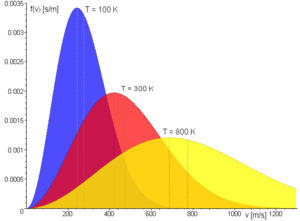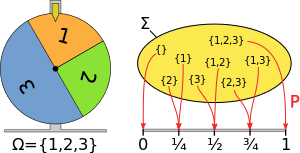# Probability measureIn some cases, statistical physics uses probability measures, but not all measures it uses are probability measures.

In mathematics, a probability measure is a real-valued function defined on a set of events in a probability space that satisfies measure properties such as countable additivity. The difference between a probability measure and the more general notion of measure (which includes concepts like area or volume) is that a probability measure must assign value 1 to the entire probability space.

Intuitively, the additivity property says that the probability assigned to the union of two disjoint events by the measure should be the sum of the probabilities of the events, e.g. the value assigned to "1 or 2" in a throw of a die should be the sum of the values assigned to "1" and "2".

Probability measures have applications in diverse fields, from physics to finance and biology.

## DefinitionA probability measure mapping the probability space for 3 events to the unit interval.

The requirements for a function μ to be a probability measure on a probability space are that:

• μ must return results in the unit interval [0, 1], returning 0 for the empty set and 1 for the entire space.
• μ must satisfy the countable additivity property that for all countable collectionsof pairwise disjoint sets:For example, given three elements 1, 2 and 3 with probabilities 1/4, 1/4 and 1/2, the value assigned to {1, 3} is 1/4 + 1/2 = 3/4, as in the diagram on the right.

The conditional probability based on the intersection of events defined as:satisfies the probability measure requirements so long asis not zero.

Probability measures are distinct from the more general notion of fuzzy measures in which there is no requirement that the fuzzy values sum up to 1, and the additive property is replaced by an order relation based on set inclusion.

## Example applications

Market measures which assign probabilities to financial market spaces based on actual market movements are examples of probability measures which are of interest in mathematical finance, e.g. in the pricing of financial derivatives. For instance, a risk-neutral measure is a probability measure which assumes that the current value of assets is the expected value of the future payoff taken with respect to that same risk neutral measure (i.e. calculated using the corresponding risk neutral density function), and discounted at the risk-free rate. If there is a unique probability measure that must be used to price assets in a market, then the market is called a complete market.

Not all measures that intuitively represent chance or likelihood are probability measures. For instance, although the fundamental concept of a system in statistical mechanics is a measure space, such measures are not always probability measures. In general, in statistical physics, if we consider sentences of the form "the probability of a system S assuming state A is p" the geometry of the system does not always lead to the definition of a probability measure under congruence, although it may do so in the case of systems with just one degree of freedom.

Probability measures are also used in mathematical biology. For instance, in comparative sequence analysis a probability measure may be defined for the likelihood that a variant may be permissible for an amino acid in a sequence.

## References

1. A course in mathematics for students of physics, Volume 2 by Paul Bamberg, Shlomo Sternberg 1991 ISBN 0-521-40650-1 page 802
2. The concept of probability in statistical physics by Yair M. Guttmann 1999 ISBN 0-521-62128-3 page 149
3. An introduction to measure-theoretic probability by George G. Roussas 2004 ISBN 0-12-599022-7 page 47
4. Probability, Random Processes, and Ergodic Properties by Robert M. Gray 2009 ISBN 1-4419-1089-1 page 163
5. Quantitative methods in derivatives pricing by Domingo Tavella 2002 ISBN 0-471-39447-5 page 11
6. Irreversible decisions under uncertainty by Svetlana I. Boyarchenko, Serge Levendorskiĭ 2007 ISBN 3-540-73745-6 page 11
7. Mathematical Methods in Biology by J. David Logan, William R. Wolesensky 2009 ISBN 0-470-52587-8 page 195
8. Discovering biomolecular mechanisms with computational biology by Frank Eisenhaber 2006 ISBN 0-387-34527-2 page 127